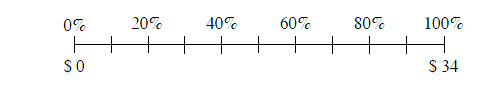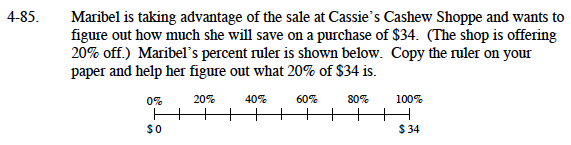### Home > MC1 > Chapter 4 > Lesson 4.3.1 > Problem4-85

4-85.

. Maribel is taking advantage of the sale at Cassie's Cashew Shoppe and wants to figure out how much she will save on a purchase of $34. (The shop is offering 20% off.) Maribel's percent ruler is shown below. Copy the ruler on your paper and help her figure out what 20% of$34 is. Homework Help ✎To start, it would be helpful to scale the lower portion of the number line. Do you remember how to do this?

Now that you know 10% is equal to $3.40, can you scale the rest of the number line to find the value of 20%? First, we can find out how much money will be just one tick mark on the number line. Start by counting the number of tick marks (10). We know that$34 will be distributed among these tick marks.

With the information gathered in Step 1, we can now find what amount will be labeled on the first tick mark (10%). We will find this value by dividing the total $34 (100%) by the number of tick marks (10). $\frac{34}{10}= \3.40$ Each tick mark represents$3.40.